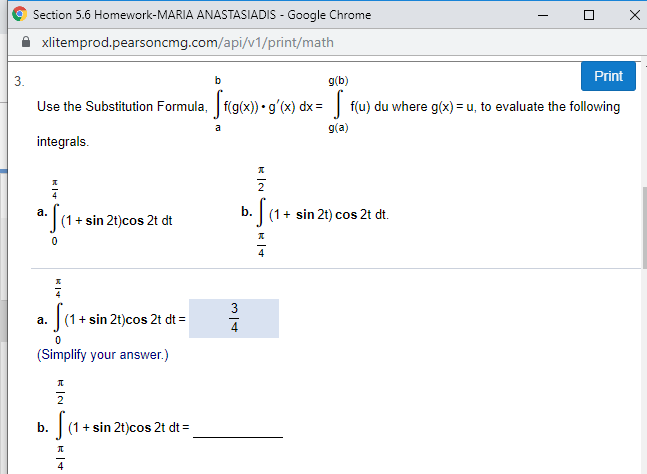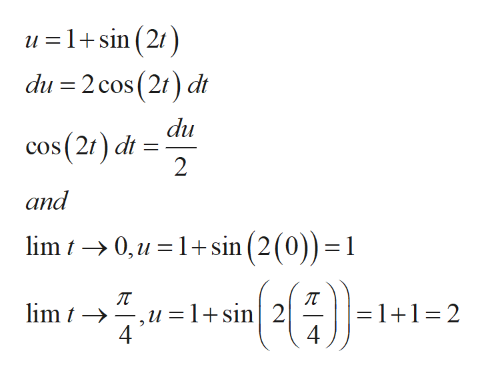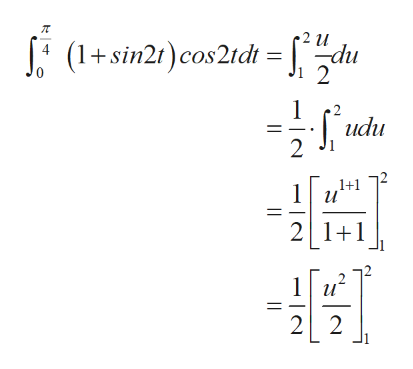# Section 5.6 Homework-MARIA ANASTASIADIS Google Chromexlitemprod.pearsoncmg.com/api/v1/print/mathPrintg(b)3.Use the Substitution Formula, f(g(x)) g'(x) dx f(u) du where g(x)= u, to evaluate the followingg(a)aintegrals2sin 2t) cos 2t dtа.(1(1+sin 2t)cos 2t dt03(1 sin 2t)cos 2t dtа.(Simplify your answer.)(1+sin 2t)cos 2t dtb.д4Пк 1ч

Question
14 views

Can you help me step by step pleasehelp_outlineImage TranscriptioncloseSection 5.6 Homework-MARIA ANASTASIADIS Google Chrome xlitemprod.pearsoncmg.com/api/v1/print/math Print g(b) 3. Use the Substitution Formula, f(g(x)) g'(x) dx f(u) du where g(x)= u, to evaluate the following g(a) a integrals 2 sin 2t) cos 2t dt а. (1 (1+sin 2t)cos 2t dt 0 3 (1 sin 2t)cos 2t dt а. (Simplify your answer.) (1+sin 2t)cos 2t dt b. д 4 П к 1ч fullscreen
check_circle

Step 1

a)

Consider the integral,

Step 2

Using substitution formula,

Lethelp_outlineImage Transcriptioncloseu =1+sin (21) du 2 cos (21) dt du cos(21) dt 2 and lim0,u 1sin (2 (0)) =1 =1+1 2 4 lim t ,u = 1+sin 2 fullscreen
Step 3

Therefore...help_outlineImage Transcriptionclose2 u =cu (1+ sin21) cos21dt 4 2 udu 2 1+1 1u 21 1 1u 2 2 fullscreen

### Want to see the full answer?

See Solution

#### Want to see this answer and more?

Solutions are written by subject experts who are available 24/7. Questions are typically answered within 1 hour.*

See Solution
*Response times may vary by subject and question.
Tagged in

### Integration